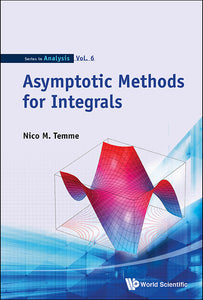# Asymptotic Methods For Integrals

Nico M Temme

Format: Print Book

ISBN: 9789814612159

• \$162.31
Unit price per
Tax included.

This book gives introductory chapters on the classical basic and standard methods for asymptotic analysis, such as Watson's lemma, Laplace's method, the saddle point and steepest descent methods, stationary phase and Darboux's method. The methods, explained in great detail, will obtain asymptotic approximations of the well-known special functions of mathematical physics and probability theory. After these introductory chapters, the methods of uniform asymptotic analysis are described in which several parameters have influence on typical phenomena: turning points and transition points, coinciding saddle and singularities. In all these examples, the special functions are indicated that describe the peculiar behavior of the integrals.The text extensively covers the classical methods with an emphasis on how to obtain expansions, and how to use the results for numerical methods, in particular for approximating special functions. In this way, we work with a computational mind: how can we use certain expansions in numerical analysis and in computer programs, how can we compute coefficients, and so on.

Format: Hardcover
No of Pages: 628
Imprint: World Scientific
Publication date: 20141103
Series: Series In Analysis